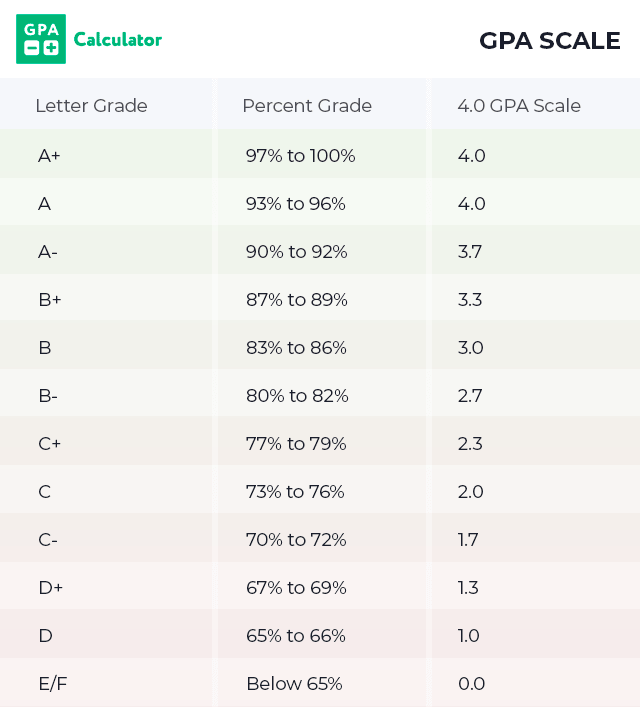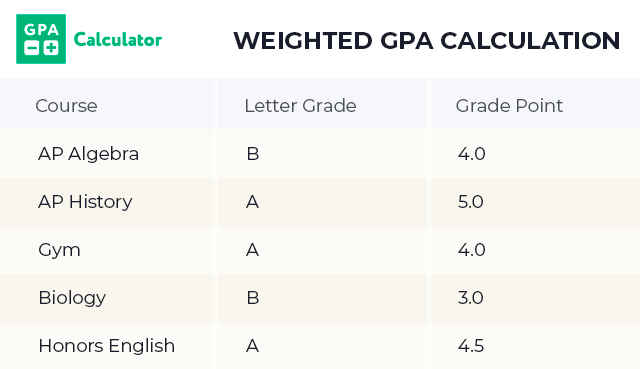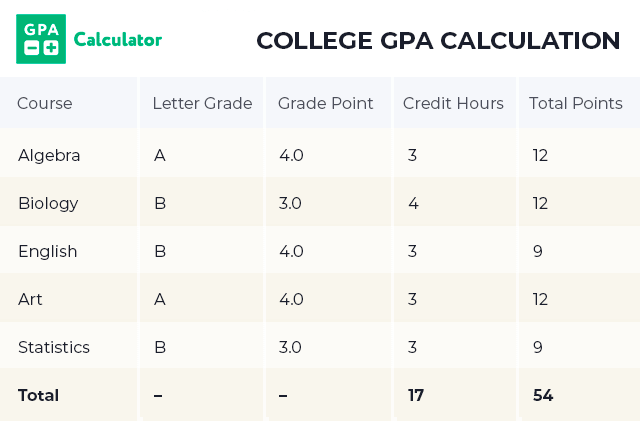# How to Calculate GPA

GPA stands for grade point average and represents the letter grades that you have earned throughout school, whether it is in college or high school. It is a cumulative score for your course grades that provides those who view it with an overall idea of how well you have done in your various classes. When a college or job looks at your GPA, they are getting an idea of the kind of student you are and how well you have grasped the concepts. If they have two applicants with the same degree, but one has a higher GPA, they know that this student did more of their homework or that they did better at grasping the concepts that were being taught to them.

## GPA Scale

When you are working to determine you GPA, the first step in the process is to turn your letter grades into the typical 4.0 scale. Each letter grade equates to a number on the scale and is used to calculate your overall GPA.GPA scales may vary a bit depending on the school, because some schools use a weighted scale that provides more points for students who are taking honors courses or AP classes. You can identify what scale your school uses and convert your letter grades to GPA scale numbers and proceed with the next step to determine your overall GPA.

The next step in the process is to find the average GPA when including all of your course. For example, if you got a 3.4 in Geometry, 3.6 in Earth Science, 3.2 in English, and 3.4 in History, you can use these numbers to determine your current GPA.

• Divide by the amount of classes

Example: 3.4 + 3.6 + 3.2 + 3.4 = 13.6 / 4 = 3.4 (89% or a B)

You can also see that if you were to bring this up just one tenth of a point, you turn that B into an A. Therefore, say in your next course you get a 3.5 or 90 %.

Example: 13.6 + 3.5 = 17.1 / 5 = 3.42 (89% or a B)

However, if you were to get a 3.9 or a 94 %

Example: 13.6 + 3.9 = 17.5 / 5 = 3.5 (90% or an A)

This is why it is so important to understand how your GPA is calculated and to know what you need to make your GPA better. Getting a 94 % in this class, can make your overall letter grade jump from a B to an A. This also increases your overall GPA.

## Weighted GPA Calculations

Some schools use a weighted GPA scale for students who have taken advanced classes. This is because these classes are designed to be more difficult than regular classes and therefore, deserve to earn the student extra grade points. Those students that take an IB or AP class get an extra full point added to their GPA for that course. Honors courses usually get an extra half point. Therefore, someone’s GPA who is taking honors or AP courses can be a full or more points higher than those taking only regular courses. This is to show that these students took harder classes. It provides a way to compare the grades of a student in regular classes with students in advanced classes.Example: 4.0 + 5.0 + 4.0 + 3.0 + 4.5 = 20.5 / 5 = 4.1 (100% or an A+)

## Calculate College GPA

When calculating your GPA in college, the credit hours for the course are included. Many of your college courses will be 3 credit courses, so there wouldn’t necessarily be a change if all of your courses are 3 credit courses. However, some courses are 4 credit hour courses. The grade points are then multiplied by the number of credit hours that the course is worth. Then the total number of points is divided by the total credit hours to calculate the overall GPA. Therefore, those courses that are worth 4 credit hours, maybe because of a lab or because the course is more difficult, provide a higher weight than the other 3 credit hour courses.To find your GPA for the semester, you would add up all of the points and then divide by your credit hours. Therefore, you would have a 3.35 GPA.

Example: 54 total points / 17 credit hours = 3.17 GPA

Then, your cumulative GPA takes your semester GPA and credit hours and then divides them by the number of semesters you have taken. This lets you know how well you have been doing overall.

When it comes to your GPA, it is important to understand how all of the factors play a role in the final number you are given. It allows you to be able to figure out what you need to do to get the GPA that you are aiming for. This is a confusing process and that is why we have provided a GPA calculator that can do all this work for you.